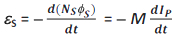Induced emf and mutual inductance

In experiment 3 discussed at the beginning of this chapter, emf is induced in coil S wherever there is any change in current through coil P. Let Φs be the flux through coil S (say of Ns turns) when current in coil P is /p . Now, flux linkages with the coil S are given by Ns Φs = M /p

If the current /p varies with time, an emf is induced in a neighbouring coil S. The induced emf is given byIt is clear that the magnitude of the induced emf depends upon the rate of change of current and mutual inductance of the two coils.

CBSE Electromagnetic Induction ( With Hint / Solution)
Class XII (By Mr. Ashis Kumar Satapathy)
email - [email protected]

Electromagnetic Induction